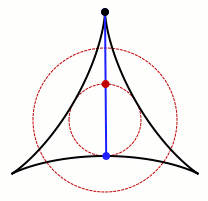## Punting in clearings of arbitrarily small Lebesgue measure

Breaking news: A £500 prize for the most elegant solution in the British Mathematical Olympiad has been founded in the memory of Christopher Bradley, whose contributions to the United Kingdom Mathematics Trust were both plentiful and invaluable. Amongst his portfolio is the bestselling Plane Euclidean Geometry (coauthored with Anthony Gardiner), which explores the interactions of algebraic curves of low degree in two dimensions from a synthetic perspective.

The advantage of this new prize is that it discourages ridiculously long case-bashes, thus making the BMO easier for us to mark. Some case-bashes are long but morally simple, such as the proof of the four-colour theorem, and it is difficult to assess whether such proofs are elegant. Nevertheless, in most cases it is easy to distinguish between an elegant solution and an inelegant one. For instance, consider the following problem:

What is the maximum number of subsets of {1, 2, 3, …, n} such that no subset is a subset of a different subset?

If we choose subsets of the same cardinality, then it’s obvious that these would satisfy the criterion. For example, with {1, 2, 3, 4} and choosing the size-2 subsets, we obtain the following six sets:

• {1, 2}
• {3, 4}
• {1, 3}
• {2, 4}
• {1, 4}
• {2, 3}

This strategy is maximised when the cardinalities of the subsets are as close to ½n as possible. It is difficult to imagine a more optimal solution, and it is a theorem (Sperner’s theorem) that this is actually the best we can manage.

Anyway, a trivial rephrasing of the problem is:

What is the maximum length of an antichain in the lattice of subsets of {1, 2, …, n}, ordered by inclusion?

By Dilworth’s theorem (a corollary of max-flow min-cut), this is equivalent to:

What is the minimum number of chains into which we can partition the lattice of subsets of {1, 2, …, n} ordered by inclusion?

We can think of the subsets of {1, 2, …, n} as being vertices of a hypercube. All of the automorphisms of the hypercube fixing the vertex corresponding to the empty set are symmetries of Sperner’s theorem.It would be nice to have a generalisation of this theorem which has all automorphisms of the hypercube as symmetries (i.e. the empty set is not given privileged status). Such a generalisation was very recently discovered by Hunter Spink in the process of proving an open problem about the maximum number of strict maxima of quadratic functions of n Boolean variables. The short but exciting paper can be found here.

So, yes, Hunter Spink is rather awesome. We were discussing the completely unrelated topic of the existence of a ‘romantic’ clearing between a Salix and a Sorbus just south of the bridge of King’s College, Cambridge. It is only just possible to punt into the clearing, perform a U-turn, and exit.

As I was describing this, Richard Freeland mentioned the well-known problem of finding the clearing with minimum area corresponding to the constraint that one can rotate a punt (considered to be a line segment) 360° within it. A reasonably good solution is the deltoid:Surprisingly, it transpires that there is no minimum area attainable. For every positive ε, we can find a simply-connected set of area < ε in which a punt can be rotated. However, the infimum of 0 cannot be obtained, i.e. there is no set of zero Lebesgue measure in which we can rotate a punt (c.f. this proof by Terence Tao). There are, however, measure-zero fractal sets obeying the strictly weaker property of containing a unit line segment in every orientation, so-called Besicovitch sets.

Generalisations to$\mathbb{R}^n$ have been considered, but this problem was found to be very difficult. As a compromise, people contemplated the analogy over finite fields: fix n > 2 and let p vary. Then, we can find a non-zero lower bound for the density of a Besicovitch set (containing a line in every direction) in the vector space$\mathbb{F}_p^n$, depending only on n and not p.

Dvir found a beautiful proof (which would certainly win Bradley’s elegance prize!), again mentioned in an article by Terry Tao.